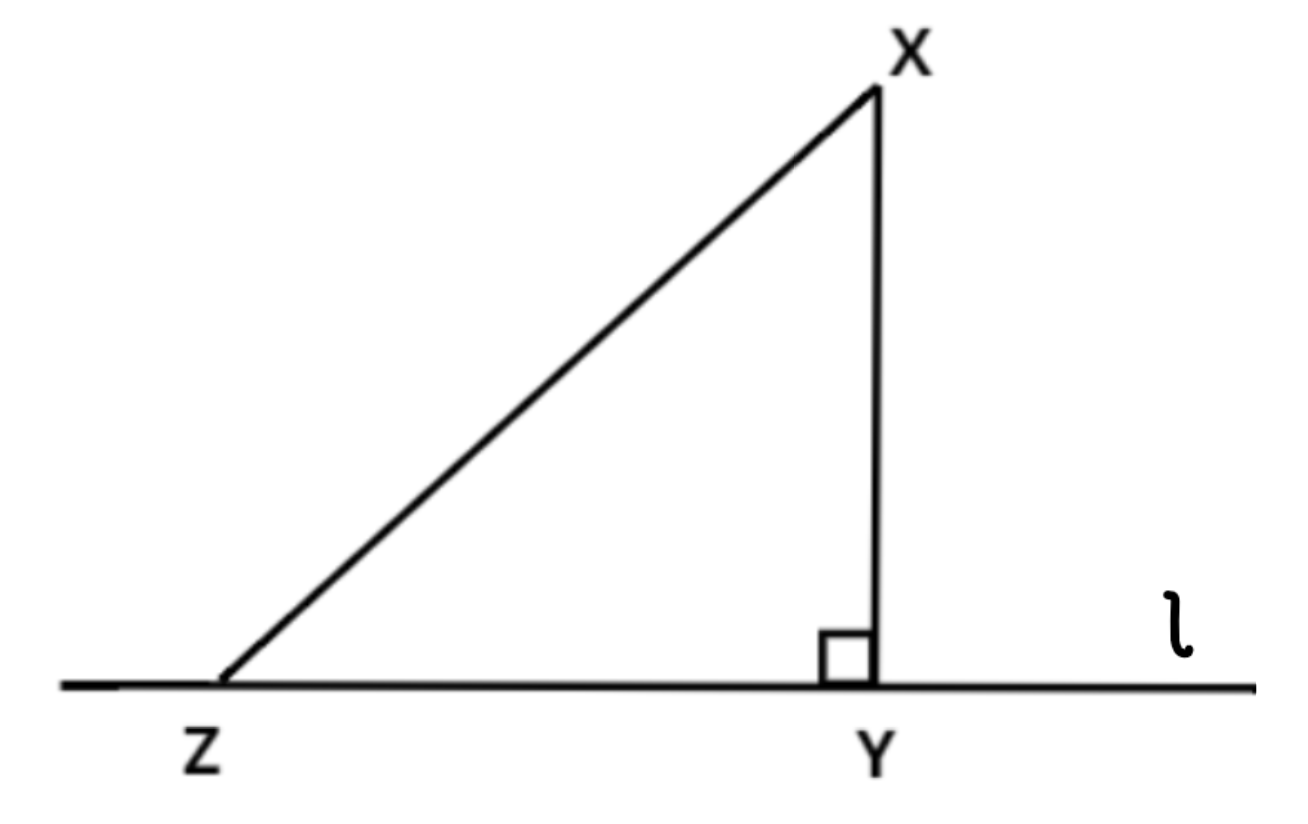Courses
Courses for Kids
Free study material
Free LIVE classes
MoreLIVE
Join Vedantu’s FREE Mastercalss

# Show that, of all line segments drawn from a given point, not on it, the perpendicular line segment is the shortest.Verified
369.3k+ views

Hint: Draw a figure and use the properties of triangles to get the necessary result.Consider a line $l$ on which two points Y and Z lie,

Now, suppose a perpendicular is drawn from a point X on the line $l$ at Y and another line segment from X meets the line $l$ at some arbitrary point Z (such that Z is not the same as Y).

To prove: XY<XZ

Proof:

Consider the $\vartriangle XYZ$

$\angle XYZ = {90^\circ} \\$

Also in $\vartriangle XYZ$

$\angle YXZ + \angle XZY + \angle XYZ = {180^\circ}$..........($\because$ Angle Sum Property)

Putting $\angle XYZ = {90^\circ} \to (1)$

We get,

$\angle YXZ + \angle XZY = {90^\circ} \to (2)$

Now, clearly $\angle XZY < {90^\circ} \to (3)$ (for the triangle to exist)

So, from equations (1) and (3), we can say that,

$\angle XZY < \angle XYZ$

So, we can say XY < XZ (side opposite to greater angle is longer)

Hence Proved.

Hence we can say that XY is the shortest compared to all line segments from point X to line $l$.

Note: Such problems can be solved by using properties of triangles like the side opposite to greater angle is the longer. Remember to draw a figure for solving more easily.

Last updated date: 28th Sep 2023
Total views: 369.3k
Views today: 8.69k